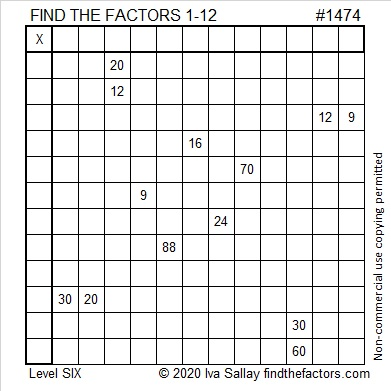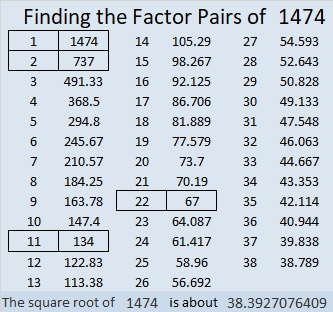# 1474 and Level 6

Contents

### Today’s Puzzle:

If you think that only kids will find the Find the Factors puzzles a challenge, then this puzzle will likely change your mind. Seriously, can you use logic to find where the factors from 1 to 12 must go to complete this puzzle? There is a reason this is a level 6 puzzle!### Factors of 1474:

The last digit of 1474 is even so 1474 is divisible by 2. Yes, that was easy, but try this next one:
1 – 4 + 7 – 4 = 0, so 1474 can be evenly divided by 11.

• 1474 is a composite number.
• Prime factorization: 1474 = 2 × 11 × 67
• 1474 has no exponents greater than 1 in its prime factorization, so √1474 cannot be simplified.
• The exponents in the prime factorization are 1, 1, and 1. Adding one to each exponent and multiplying we get (1 + 1)(1 + 1)(1 + 1) = 2 × 2 × 2 = 8. Therefore 1474 has exactly 8 factors.
• The factors of 1474 are outlined with their factor pair partners in the graphic below.### Another Fact about the Number 1474:

1474 can not be written as the sum of two squares or the difference of two squares, but it is still the short leg in two Pythagorean triples. Here is one of them:
1474-4368-4610, calculated from 2(67)(11), 67² – 11², 67² + 11²

This site uses Akismet to reduce spam. Learn how your comment data is processed.